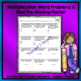# 3rd Grade: Operations and Algebraic Thinking WorksheetSubject
Resource Type
File Type

PDF

Standards
• Product Description
• StandardsNEW

Do your scholars struggle with some of the skills in 3.OA? Well, this no prep resource is aligned to the Common Core 3.OA standards and will allow your scholars to practice, review, as well as prep for the state test. Teacher...rest assured your scholars will be challenged with this resource. It contains level 1 - 3 questions.

Print - Engage - Learn!!

Question Types:

Selected response

Multi-Selected

Constructed Response

Part A/B/C

Included In This Resource:

9 practice pages

FEEDBACK

I truly value your feedback. Earn each time you provide feedback!! TPT gives you TPT credit you can use toward lowering the cost of other products.

I would love to send you notifications of sales, new product launches, and freebies.

Click on the GREEN star next to my logo, to follow me. New products are always 50% off for the 1st 24 hours they are posted. Follow me... it pays!!

Relish in Teaching,

Priscilla A. Woodard

Identify arithmetic patterns (including patterns in the addition table or multiplication table), and explain them using properties of operations. For example, observe that 4 times a number is always even, and explain why 4 times a number can be decomposed into two equal addends.
Solve two-step word problems using the four operations. Represent these problems using equations with a letter standing for the unknown quantity. Assess the reasonableness of answers using mental computation and estimation strategies including rounding.
Fluently multiply and divide within 100, using strategies such as the relationship between multiplication and division (e.g., knowing that 8 × 5 = 40, one knows 40 ÷ 5 = 8) or properties of operations. By the end of Grade 3, know from memory all products of two one-digit numbers.
Understand division as an unknown-factor problem. For example, find 32 ÷ 8 by finding the number that makes 32 when multiplied by 8.
Apply properties of operations as strategies to multiply and divide. Examples: If 6 × 4 = 24 is known, then 4 × 6 = 24 is also known. (Commutative property of multiplication.) 3 × 5 × 2 can be found by 3 × 5 = 15, then 15 × 2 = 30, or by 5 × 2 = 10, then 3 × 10 = 30. (Associative property of multiplication.) Knowing that 8 × 5 = 40 and 8 × 2 = 16, one can find 8 × 7 as 8 × (5 + 2) = (8 × 5) + (8 × 2) = 40 + 16 = 56. (Distributive property.)
Total Pages
Included
Teaching Duration
N/A
Report this Resource to TpT
Reported resources will be reviewed by our team. Report this resource to let us know if this resource violates TpT’s content guidelines.